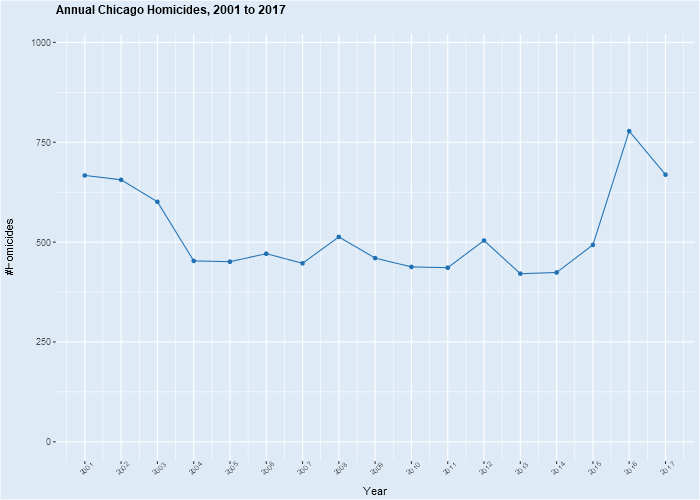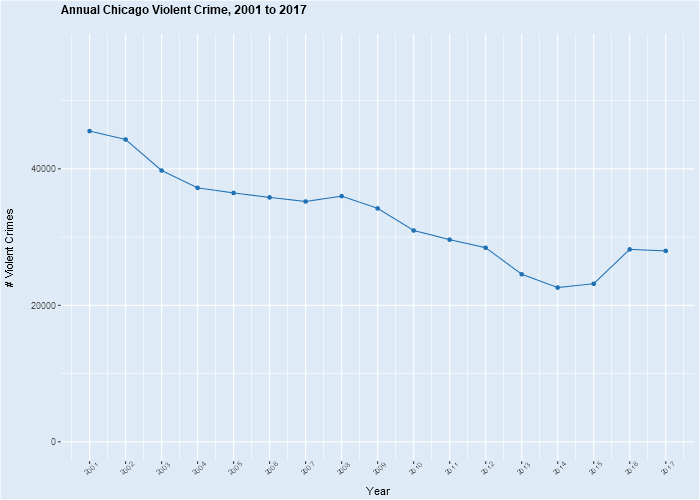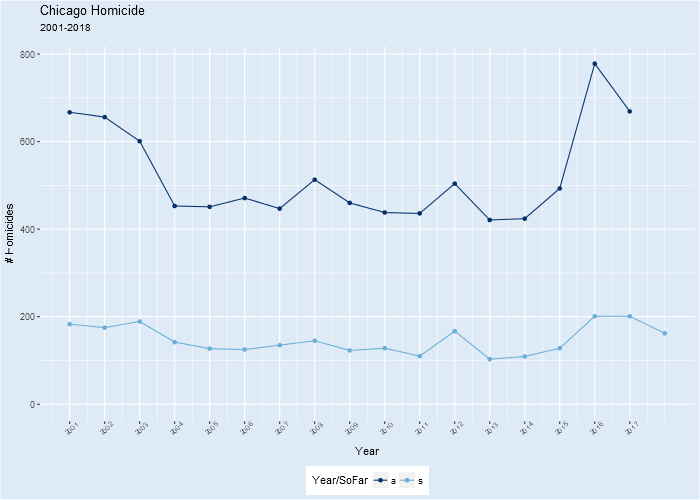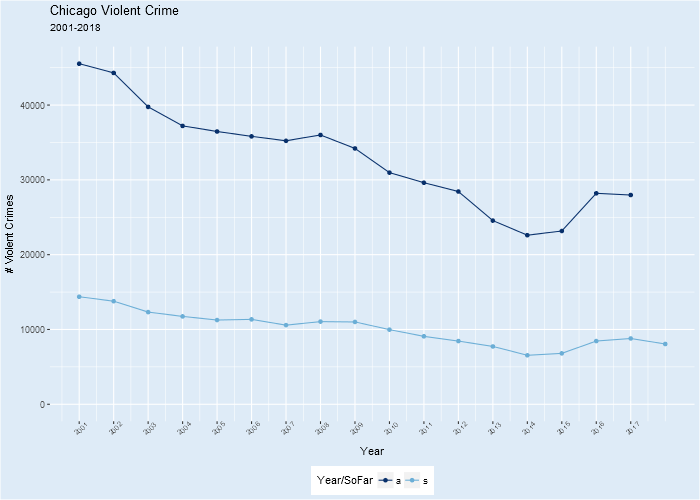### Frequencies in Pandas -- and a Little R Magic for Python¶

I've got a big digital mouth. Last time, I wrote on frequencies using R, noting cavalierly that I'd done similar development in Python/Pandas. I wasn't lying, but the pertinent work I dug up from two years ago was less proof and more concept.

Of course, R and Python are the two current language leaders for data science computing, while Pandas is to Python as data.table and tidyverse are to R for data management: everything.

So I took on the challenge of extending the work I'd started in Pandas to replicate the frequencies functionality I'd developed in R. I was able to demonstrate to my satisfaction how it might be done, but not before running into several pitfalls.

Pandas is quite the comprehensive library, aiming "to be the fundamental high-level building block for doing practical, real world data analysis in Python." I think it succeeds, providing highly-optimized structures for efficiently managing/analyzing data. The primary Pandas data structures are the series and the dataframe; the Pandas developer mainly uses core Python to manage these structures.

Pandas provides a procedure, value_counts(), to output frequencies from a series or a single dataframe column. To include null or NA values, the programmer designates dropna=False in the function call.

Alas, value_counts() works on single attributes only, so to handle the multi-variable case, the programmer must dig into Pandas's powerful split-apply-combine groupby functions. There is a problem with this though: by default, these groupby functions automatically delete NA's from consideration, even as it's generally the case with frequencies that NA counts are desirable. What's the Pandas developer to do?

There are several work-arounds that can be deployed. The first is to convert all groupby "dimension" vars to string, in so doing preserving NA's. That's a pretty ugly and inefficient band-aid, however. The second is to use the fillna() function to replace NA's with a designated "missing" value such as 999.999, and then to replace the 999.999 later in the chain with NA after the computations are completed. I'd gone with the string conversion option when first I considered frequencies in Pandas. This time, though, I looked harder at the fillna-replace option, generally finding it the lesser of two evils.

The remainder of this notebook looks at these Pandas frequencies options for the same Chicago crime data with almost 6.6M records I illustrated last time. I first build a working data set from the downloaded csv file, then take a look at the different options noted above, finally settling on a poc frequency function using fillna-replace.

Gratuitously, I also demo rmagic from the rpy2 Python package that allows R capabilities to be included in a Python program, much as the R package reticulate does in the other direction. Both rpy2 and reticulate are harbingers of soon-coming inclusive interoperability between R and Python. That's all good for data scientists!

Import a few Python libraries.

In :
import warnings, os, sys, numpy as np, pandas as pd, time, datetime
warnings.filterwarnings('ignore')

print("\n\n")





Load the rpy2 "magic" R extension

In :
%load_ext rpy2.ipython

print("\n\n")

The rpy2.ipython extension is already loaded. To reload it, use:
%reload_ext rpy2.ipython



Import other rpy2 libraries and activate the the Pandas to R dataframe copy capability for later use.

In :
import rpy2
import rpy2.robjects.numpy2ri
import rpy2.robjects as robjects
from robjects import r, pandas2ri

pandas2ri.activate()

print("\n\n")





Define a few simple lambda functions.

In :
pr2 = lambda : print("\n\n")

tally = lambda df:range(len(df))

pr2()





Assign directories and file names.

In :
dir = "c:/bigdata/raw/chicago"
os.chdir(dir)

cname = "communities.csv"
crname = "chicagocrime.csv"
fname = "fbicode.csv"

MISSING = "-999.999-"

pr2()





Read the Chicago communities data file into a Pandas dataframe.

In :
colnames = open(cname, 'r').readline().split(",")
communities = pd.read_csv(cname, header=0,skiprows=1,dtype={'GeogKey':np.float64})
communities.columns = [c.replace("\n",'').replace('*','').replace(' ', '').lower() for c in colnames]
communities = communities[['geogkeyx', 'geogname','p0050001']]

communities.columns = ['communityarea', 'name','population']

print(communities.info())

pr2()

<class 'pandas.core.frame.DataFrame'>
RangeIndex: 77 entries, 0 to 76
Data columns (total 3 columns):
communityarea    77 non-null float64
name             77 non-null object
population       77 non-null int64
dtypes: float64(1), int64(1), object(1)
memory usage: 1.9+ KB
None



Ditto for the FBI crime code/description file.

In :
fbicode = pd.read_csv(fname)

print(fbicode.info())

pr2()

<class 'pandas.core.frame.DataFrame'>
RangeIndex: 26 entries, 0 to 25
Data columns (total 2 columns):
fbicode        26 non-null object
fbicodedesc    26 non-null object
dtypes: object(2)
memory usage: 496.0+ bytes
None



Read several attributes from the latest Chicago crime csv file downloaded in a separate process. Join in the communities and fbicode descriptions to make crimeplus, the final Pandas dataframe of almost 6.6M records. The unexecuted code between the two """s, illustrates how to make categorical variables from character objects. The "null" list tells which columns have NA's.

In :
start = time.time()

hdr = pd.read_csv(crname,nrows=0,header=0)
nmes = [h.replace('*','').replace(' ', '').lower() for h in hdr.columns]

vars = ['date','communityarea','fbicode','latitude','longitude']

crime =  pd.read_csv(crname,header=None,names=nmes,usecols=vars,skiprows=1,
dtype={'communityarea':np.float64},index_col=False)

crime.date = pd.to_datetime(crime.date,format='%m/%d/%Y %H:%M:%S %p')

crimeplus = pd.merge(pd.merge(crime[vars], communities, how='left',
on='communityarea'),fbicode,how='left',on='fbicode')

"""
crimeplus.fbicode = pd.Categorical(crimeplus.fbicode)
crimeplus.fbicodedesc = pd.Categorical(crimeplus.fbicodedesc)
crimeplus.communityarea = pd.Categorical(crimeplus.communityarea)
crimeplus.name = pd.Categorical(crimeplus.name)

crimeplus.fbicode = crimeplus.fbicode.cat.add_categories(MISSING)
crimeplus.fbicodedesc = crimeplus.fbicodedesc.cat.add_categories(MISSING)
crimeplus.communityarea = crimeplus.communityarea.cat.add_categories(MISSING)
crimeplus.name = crimeplus.name.cat.add_categories(MISSING)
"""

null = crimeplus.columns[crimeplus.isnull().any()]
print(null,"\n")

end = time.time()
print(end-start,"\n")

print (crimeplus.info(),"\n")

pr2()

Index(['communityarea', 'latitude', 'longitude', 'name', 'population'], dtype='object')

45.03502345085144

<class 'pandas.core.frame.DataFrame'>
Int64Index: 6599283 entries, 0 to 6599282
Data columns (total 8 columns):
date             datetime64[ns]
communityarea    float64
fbicode          object
latitude         float64
longitude        float64
name             object
population       float64
fbicodedesc      object
dtypes: datetime64[ns](1), float64(4), object(3)
memory usage: 453.1+ MB
None



Define categories of crime from the fbicodes.

In :
homicide = set(["01A"])
violentcrime = set(["01A","01B","02","03","04A","04B"])
propertycrime = set(["05","06","07","09"])
indexcrime = set(['01A','01B','02','03','04A','04B','05','06','07','09'])

pr2()





Compute frequencies for the communityarea attribute in the crimeplus dataframe using the Pandas value_counts() function, specifying the inclusion of NA's.

In :
freqs = crimeplus.communityarea.value_counts(dropna=False)
print(freqs.head(),"\n")

print(crimeplus.communityarea.isnull().sum())

pr2()

NaN      616029
25.0    382409
8.0     201625
43.0    195319
23.0    189500
Name: communityarea, dtype: int64

616029



Compute a bivariate frequencies data.frame with NA's using the workaround of converting the frequency variables to string first, then computing the frequencies, and finally transforming the frequency table columns back to their original types. This sequence is slow and klunky.

In :
fvar = ['communityarea','fbicodedesc']

freqss = crimeplus[fvar].copy().astype(str).groupby(fvar).size().reset_index()

freqss.columns = fvar + ['frequency']

freqss.communityarea = freqss.communityarea.astype(np.float64)

freqss.sort_values('frequency',ascending=False, inplace=True)

freqss.index = tally(freqss)

print(freqss.head(),"\n")
print(freqss.frequency[freqss.communityarea.isnull()].sum(),"\n")
print(freqss.info(),"\n")

pr2()

   communityarea     fbicodedesc  frequency
0            NaN         Larceny     123083
1            NaN  Simple Battery      98329
2            8.0         Larceny      83536
3           25.0      Drug Abuse      82085
4           32.0         Larceny      71827

616029

<class 'pandas.core.frame.DataFrame'>
RangeIndex: 1981 entries, 0 to 1980
Data columns (total 3 columns):
communityarea    1956 non-null float64
fbicodedesc      1981 non-null object
frequency        1981 non-null int64
dtypes: float64(1), int64(1), object(1)
memory usage: 46.5+ KB
None



Now do the same computation using the alternative workaround of first converting NA's to a designated "MISSING", then computing the frequencies, and finally replacing the MISSING's with NA's. This is a simpler option that performs better.

In :
fvar = ['communityarea','fbicodedesc']

freqsf = crimeplus[fvar].fillna(MISSING).groupby(fvar).size().reset_index().replace(MISSING,np.NaN)
freqsf.columns = fvar + ['frequency']

freqsf.sort_values('frequency',ascending=False,inplace=True)
freqsf.index = tally(freqsf)

print(freqsf.head(),"\n")

print(freqsf.frequency[freqsf.communityarea.isnull()].sum(),"\n")

print(freqsf.info())

pr2()

   communityarea     fbicodedesc  frequency
0            NaN         Larceny     123083
1            NaN  Simple Battery      98329
2            8.0         Larceny      83536
3           25.0      Drug Abuse      82085
4           32.0         Larceny      71827

616029

<class 'pandas.core.frame.DataFrame'>
RangeIndex: 1981 entries, 0 to 1980
Data columns (total 3 columns):
communityarea    1956 non-null float64
fbicodedesc      1981 non-null object
frequency        1981 non-null int64
dtypes: float64(1), int64(1), object(1)
memory usage: 46.5+ KB
None



Define a generic frequencies function prototype, using the "MISSING" approach.

In :
def frequenciesf(df,fvar):

freqs = df[fvar].fillna(MISSING).groupby(fvar).size().reset_index().replace(MISSING,np.NaN)
freqs.columns = fvar + ['frequency']
N = freqs.frequency.sum()
freqs['percent']  = 100.0*freqs.frequency.astype(float)/N

freqs.sort_values(['frequency']+fvar, ascending=False, inplace=True)
freqs.index = tally(freqs)

return(freqs)

pr2()





Test it first on a single attribute.

In :
freqs = frequenciesf(crimeplus,['communityarea'])
print(freqs.head(),"\n")
print(crimeplus.communityarea.isna().sum())

pr2()

   communityarea  frequency   percent
0            NaN     616029  9.334787
1           25.0     382409  5.794705
2            8.0     201625  3.055256
3           43.0     195319  2.959700
4           23.0     189500  2.871524

616029



Now consider a 3-way frequencies combination with the new function. The performance isn't bad.

In :
fvar = ["communityarea",'population',"fbicodedesc"]

start = time.time()
freqsf = frequenciesf(crimeplus,fvar)
end = time.time()
print(end-start,"\n")

print(freqsf.head())

pr2()

4.537893533706665

communityarea  population     fbicodedesc  frequency   percent
0            NaN         NaN         Larceny     123083  1.865097
1            NaN         NaN  Simple Battery      98329  1.489995
2            8.0     80484.0         Larceny      83536  1.265834
3           25.0     98514.0      Drug Abuse      82085  1.243847
4           32.0     29283.0         Larceny      71827  1.088406



A few more examples -- the first subsetting for homicide and partial years. Note the addition followed by deletion of the computed year attribute from the data.frame.

In :
myear = crimeplus.date.dt.year.max()

numdays = crimeplus[crimeplus.date.dt.year==myear].date.dt.dayofyear.max()

maxdt = crimeplus.date.dt.date.max()
print(maxdt)
print(numdays)

crimeplus['year'] = crimeplus.date.dt.year

fvar = ['year']

freqs = frequenciesf(crimeplus[(crimeplus.fbicode.isin(homicide)) &
(crimeplus.date.dt.dayofyear<=numdays)],fvar)
print(freqs,"\n")
print(freqs.info())

crimeplus.drop(['year'], axis=1, inplace=True)

pr2()

2018-05-08
128
year  frequency   percent
0   2017        201  7.576329
1   2016        201  7.576329
2   2003        189  7.124011
3   2001        183  6.897851
4   2002        175  6.596306
5   2012        167  6.294761
6   2018        162  6.106295
7   2008        145  5.465511
8   2004        142  5.352431
9   2007        135  5.088579
10  2015        128  4.824727
11  2010        128  4.824727
12  2005        127  4.787034
13  2006        125  4.711647
14  2009        123  4.636261
15  2011        110  4.146250
16  2014        109  4.108556
17  2013        103  3.882397

<class 'pandas.core.frame.DataFrame'>
RangeIndex: 18 entries, 0 to 17
Data columns (total 3 columns):
year         18 non-null int64
frequency    18 non-null int64
percent      18 non-null float64
dtypes: float64(1), int64(2)
memory usage: 512.0 bytes
None



Take a look at NA's for communityarea by longitude.

In :
fvar = ['isna_communityarea','isna_longitude']

crimeplus[fvar] = crimeplus.communityarea.isnull()
crimeplus[fvar] = crimeplus.longitude.isnull()

print(crimeplus['isna_communityarea'].sum(),"\n")

freqs = frequenciesf(crimeplus,fvar)

print(freqs,"\n")
print(freqs.info())

crimeplus.drop(fvar, axis=1, inplace=True)

pr2()

616029

isna_communityarea  isna_longitude  frequency    percent
0               False           False    5934460  89.925830
1                True           False     606670   9.192968
2               False            True      48794   0.739383
3                True            True       9359   0.141818

<class 'pandas.core.frame.DataFrame'>
RangeIndex: 4 entries, 0 to 3
Data columns (total 4 columns):
isna_communityarea    4 non-null bool
isna_longitude        4 non-null bool
frequency             4 non-null int64
percent               4 non-null float64
dtypes: bool(2), float64(1), int64(1)
memory usage: 152.0 bytes
None



Now consider homicides by month and year.

In :
fvar = ['year','month']

crimeplus[fvar] = crimeplus.date.dt.year
crimeplus[fvar] = crimeplus.date.dt.month

freqs = frequenciesf(crimeplus[crimeplus.fbicode.isin(homicide)],fvar)

print(freqs.head(10),"\n")
print(freqs.info())

crimeplus.drop(fvar, axis=1, inplace=True)

pr2()

   year  month  frequency   percent
0  2016      8         95  1.050420
1  2017      6         86  0.950907
2  2016     10         82  0.906678
3  2016     11         79  0.873507
4  2016      6         78  0.862450
5  2001      7         78  0.862450
6  2002      8         77  0.851393
7  2017      7         76  0.840336
8  2001     10         71  0.785051
9  2001      9         71  0.785051

<class 'pandas.core.frame.DataFrame'>
RangeIndex: 209 entries, 0 to 208
Data columns (total 4 columns):
year         209 non-null int64
month        209 non-null int64
frequency    209 non-null int64
percent      209 non-null float64
dtypes: float64(1), int64(3)
memory usage: 6.6 KB
None



Another look at homicides by year followed by a little rmagic -- passing the frequencies dataframe to R for ggplot.

In :
myear = crimeplus.date.dt.year.max()

crimeplus['year'] = crimeplus.date.dt.year

fvar = ['year']

freqs = frequenciesf(crimeplus[(crimeplus.fbicode.isin(homicide))
& (crimeplus.year<myear)],fvar)

print(freqs)

crimeplus.drop(['year'], axis=1, inplace=True)

rfreqs = pandas2ri.py2ri(freqs)

pr2()

    year  frequency   percent
0   2016        778  8.759288
1   2017        669  7.532087
2   2001        667  7.509570
3   2002        656  7.385724
4   2003        601  6.766494
5   2008        513  5.775726
6   2012        504  5.674398
7   2015        493  5.550552
8   2006        471  5.302860
9   2009        460  5.179014
10  2004        453  5.100203
11  2005        451  5.077685
12  2007        447  5.032650
13  2010        438  4.931322
14  2011        436  4.908804
15  2014        424  4.773700
16  2013        421  4.739923



Using "rmagic", load pertinent R libraries and use ggplot on the frequencies data.frame.

In :
%R require(ggplot2); require(tidyr); require(data.table); require(RColorBrewer);require(R.utils)

Out:
array(, dtype=int32)
In :
%%R  -w700 -h500 -i rfreqs

murders <- data.table(year=as.integer(as.character(rfreqs$year)),count=rfreqs$frequency)#[year<2018]

pal <- brewer.pal(9,"Blues")

ggplot(murders,aes(x=year,y=count,col=pal)) +
geom_point() +
geom_line() +
theme(legend.position = "none", plot.background = element_rect(fill = pal),
panel.background = element_rect(fill = pal)) +
ylim(0,max(murders$count)*1.25) + theme(axis.text.x = element_text(size=7, angle = 45)) + theme(legend.position="none") + scale_color_manual(values=pal) + scale_x_continuous(breaks=sort(unique(murders$year))) +
theme(plot.title = element_text (face="bold",size=12)) +
labs(title=paste("Annual Chicago Homicides, ",min(murders$year), " to ", max(murders$year),"\n",sep=""),x="Year",y="#Homicides\n")The same with violentcrime.

In :
myear = crimeplus.date.dt.year.max()

crimeplus['year'] = crimeplus.date.dt.year

fvar = ['year']

freqs = frequenciesf(crimeplus[(crimeplus.fbicode.isin(violentcrime))
& (crimeplus.year<myear)],fvar)

print(freqs)

crimeplus.drop(['year'], axis=1, inplace=True)

rfreqs = pandas2ri.py2ri(freqs)

pr2()

    year  frequency   percent
0   2001      45531  8.130202
1   2002      44296  7.909675
2   2003      39755  7.098816
3   2004      37213  6.644906
4   2005      36466  6.511518
5   2008      36003  6.428843
6   2006      35815  6.395273
7   2007      35220  6.289027
8   2009      34203  6.107428
9   2010      30967  5.529594
10  2011      29620  5.289068
11  2012      28438  5.078006
12  2016      28203  5.036043
13  2017      27976  4.995509
14  2013      24552  4.384106
15  2015      23164  4.136259
16  2014      22601  4.035727


In :
%%R  -w700 -h500 -i rfreqs

violent <- data.frame(year=as.integer(as.character(rfreqs$year)),count=rfreqs$frequency)#[year<2018]

pal <- brewer.pal(9,"Blues")

ggplot(violent,aes(x=year,y=count,col=pal)) +
geom_point() +
geom_line() +
theme(legend.position = "none", plot.background = element_rect(fill = pal),
panel.background = element_rect(fill = pal)) +
ylim(0,max(violent$count)*1.25) + theme(axis.text.x = element_text(size=7, angle = 45)) + theme(legend.position="none") + scale_color_manual(values=pal) + scale_x_continuous(breaks=sort(unique(violent$year))) +
theme(plot.title = element_text (face="bold",size=12)) +
labs(title=paste("Annual Chicago Violent Crime, ",min(violent$year), " to ", max(violent$year),"\n",sep=""),
x="Year",y="# Violent Crimes\n")Now juxtapose annual homicides with those through "numdays" (128) of the year.

In :
myear = crimeplus.date.dt.year.max()

numdays = crimeplus[crimeplus.date.dt.year==myear].date.dt.dayofyear.max()

print(numdays)

crimeplus['year'] = crimeplus.date.dt.year

fvar = ['year']

vars = ['year','frequency']
freqs1 = frequenciesf(crimeplus[(crimeplus.fbicode.isin(homicide)) &
(crimeplus.date.dt.dayofyear<=numdays)],fvar)[vars].sort_values(['year'])
freqs2 = frequenciesf(crimeplus[crimeplus.fbicode.isin(homicide)],fvar)[vars].sort_values(['year'])

freqs1.columns = ['year','freq']
freqs2.columns = ['year','freq']
freqs1['which'] = "s"
freqs2['which'] = "a"

freqs3 = freqs1.append(freqs2)
mxyear = freqs3.year.max()

freqs3.freq[(freqs3.year==mxyear) & (freqs3.which=='a')]  = np.NaN
freqs3.index = tally(freqs3)

print(freqs3.head(),"\n")
print(freqs3.tail(),"\n")

crimeplus.drop(['year'], axis=1, inplace=True)

rfreqs3 = pandas2ri.py2ri(freqs3)

pr2()

128
year   freq which
0  2001  183.0     s
1  2002  175.0     s
2  2003  189.0     s
3  2004  142.0     s
4  2005  127.0     s

year   freq which
31  2014  424.0     a
32  2015  493.0     a
33  2016  778.0     a
34  2017  669.0     a
35  2018    NaN     a


In :
%%R  -w700 -h500 -i rfreqs3

pal <- brewer.pal(9,"Blues")

ggplot(rfreqs3,aes(x=year,y=freq,col=which)) +
geom_point() +
geom_line() +
theme(legend.position = "bottom", plot.background = element_rect(fill = pal),
panel.background = element_rect(fill = pal)) +
ylim(0,max(rfreqs3$freq)*1.25) + scale_color_manual(values=pal[c(9,5)]) + theme(axis.text.x = element_text(size=7, angle = 45)) + scale_x_continuous(breaks=sort(unique(rfreqs$year))) +
labs(title="Chicago Homicide", subtitle="2001-2018\n",
x="Year", y="# Homicides", color="Year/SoFar")Ditto for violent crime.

In :
myear = crimeplus.date.dt.year.max()

numdays = crimeplus[crimeplus.date.dt.year==myear].date.dt.dayofyear.max()

print(numdays)

crimeplus['year'] = crimeplus.date.dt.year

fvar = ['year']

vars = ['year','frequency']
freqs1 = frequenciesf(crimeplus[(crimeplus.fbicode.isin(violentcrime)) &
(crimeplus.date.dt.dayofyear<=numdays)],fvar)[vars].sort_values(['year'])
freqs2 = frequenciesf(crimeplus[crimeplus.fbicode.isin(violentcrime)],fvar)[vars].sort_values(['year'])

freqs1.columns = ['year','freq']
freqs2.columns = ['year','freq']
freqs1['which'] = "s"
freqs2['which'] = "a"

freqs3 = freqs1.append(freqs2)#.reset_index()
freqs3.index = tally(freqs3)
mxyear = freqs3.year.max()

freqs3.freq[(freqs3.year==mxyear) & (freqs3.which=='a')]  = np.NaN
print(freqs3.head())

crimeplus.drop(['year'], axis=1, inplace=True)

rfreqs3 = pandas2ri.py2ri(freqs3)

pr2()

128
year     freq which
0  2001  14377.0     s
1  2002  13779.0     s
2  2003  12322.0     s
3  2004  11744.0     s
4  2005  11259.0     s


In :
%%R  -w700 -h500 -i rfreqs3

pal <- brewer.pal(9,"Blues")

ggplot(rfreqs3,aes(x=year,y=freq,col=which)) +
geom_point() +
geom_line() +
theme(legend.position = "bottom", plot.background = element_rect(fill = pal),
panel.background = element_rect(fill = pal)) +
ylim(0,max(rfreqs3$freq)*1.25) + scale_color_manual(values=pal[c(9,5)]) + theme(axis.text.x = element_text(size=7, angle = 45)) + scale_x_continuous(breaks=sort(unique(rfreqs$year))) +
labs(title="Chicago Violent Crime", subtitle="2001-2018\n",
x="Year", y="# Violent Crimes", color="Year/SoFar")That's it for now. More R-reticulate/Pandas-rpy2/ later.